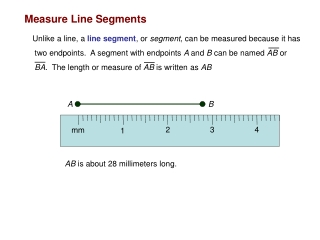DownloadDownload PresentationMeasure Line Segments

# Measure Line Segments

Download Presentation## Measure Line Segments

- - - - - - - - - - - - - - - - - - - - - - - - - - - E N D - - - - - - - - - - - - - - - - - - - - - - - - - - -
##### Presentation Transcript

1. Measure Line Segments Unlike a line, a line segment, or segment, can be measured because it has two endpoints. A segment with endpoints A and B can be named AB or BA. The length or measure of AB is written as AB A B 2 3 4 mm 1 AB is about 28 millimeters long.

2. Calculate Measures Measures are real numbers, so all arithmetic operations can be used with them. We know that the whole usually equals the sum of its parts. This is also true of line segments in geometry. Betweenness of Points Point M is between points P and Q if and only if P, Q, and M are collinear and PM + MQ = PQ Q M P

3. Example 1 - Find Measurements 1. Find AC AC is the measure of AC A 3.3 cm B 3.4 cm C AC can be found by adding AB and BC AB + BC = AC Sum of the parts = whole 3.3 + 3.4 = AC Substitution 6.7 = ACAdd

4. Example 2 - Find Measurements 2. Find DE DE is the measure of DE F 3 E D 12 AC can be found by adding AB and BC DE + EF = DF Sum of the parts = whole DE+ 3 = 12Substitution DE+ 3 - 3 = 12 - 3 Subtract 3 from each side DE = 9 Simplify

5. Example 3 - Find Measurements 3. Find y and PQ if P is between Q and R, PQ = 2y, QR = 3y + 1, and PR = 21 3y + 1 21 2y Q P R QR = QP + PR 3y + 1 = 2y + 21Substitute known values 3y + 1 -1 = 2y + 21 – 1Subtract one from each side 3y = 2y + 20Simplify 3y – 2y = 2y + 20 – 2y Subtract 2y from each side y = 20 Simplify PQ = 2yGiven PQ = 2(20)y = 20 PQ = 40 multiply

6. Y X P Q

7. Example 4 - Find Measurements Find RS and ST 6x - 5 2x + 3 R S T 30

8. Example 5 - Find Measurements Find a, RT 7a 12a R S T 28

9. Example 6 - Find Measurements Find x, RS and ST 2x 3x R S T 25

10. Example 7 - Find Measurements Find x, RS and ST 3y + 1 2y R S T 21

11. Example 8 - Find Measurements Find x, RT and ST 12 2x R S T 5x + 10

12. Example 9 - Find Measurements Find y, RS and ST 4y - 1 2y - 1 R S T 5y